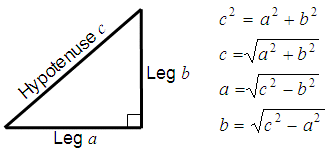# Pythagorean Theorem Calculator

Basics

Examples

Online Calculator

### Basics

This is a calculator for the Phytagorean Theorem. Given the two legs of a right triangle (legs a and b), you can calculate the hypotenuse (c); or, if you're given the hypotenuse (c) and a leg (either a or b), you can find the other leg of the right triangle.

A corollary of the theorem is that in any right triangle, the hypotenuse is greater than any one of the legs, but less than the sum of them.### Examples - Pythagoras' Triangle

Example 1:

If one leg of a right triangle is 3 inches and the other leg is 4, what's the length of the hypotenuse?

You can enter into the calculator
Leg a = 3
Leg b = 4

and you get

Hypotenuse c = 5

Example 2:

If the hypotenuse of a triangle is 10 ft and a leg is 6 ft, how long is the other leg of the triangle?

You can enter
Hypotenuse c = 10
Leg a = 6

and you get

Leg b = 8

### Online Calculator

To be able to use the following calculator you have to allow the use of JavaScript codes on your navigator.

 Enter the two legs of a right triangle to get the hypotenuse... Leg a = Leg b = Hypotenuse = or enter any leg and the hypotenuse to get the other leg of the triangle... Any leg = Hypotenuse = Other leg =